This is a Clilstore unit. You can .

# List of Geometric Shapes

2d Geometric Shapes

Here are our list of 2d geometric shapes, including triangles, quadrilaterals and polygons

List of Geometric Shapes - Triangles

 Equilateral Triangle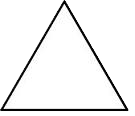Equilateral triangles have all angles equal to 60° and all sides equal length. All equilateral triangles have 3 lines of symmetry. Isoscles Triangle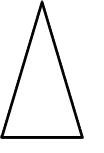Isosceles triangles have 2 angles equal and 2 sides of equal length. All isosceles triangles have a line of symmetry. Scalene TriangleScalene triangles have no angles equal, and no sides of equal length. Right Triangle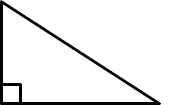Right triangles (or right angled triangles) have one right angle (equal to 90° ). Obtuse Triangle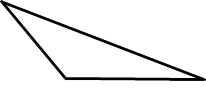Obtuse triangles have one obtuse angle (an angle greater than 90° ). The other two angles are acute (less than 90° ). Acute Triangle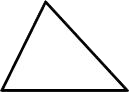Acute triangles have all angles acute.

List of Geometric Shapes - Quadrilaterals

A quadrilateral is a polygon with 4 sides.

Quadrilaterals are also sometimes called quadrangles or tetragons.

 Square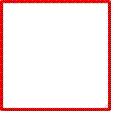Squares have 4 equal sides and 4 right angles. They have 4 lines of symmetry. All squares belong to the rectangle family. All squares belong to the rhombus family. All squares are also parallelograms. Rectangle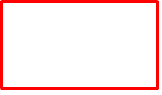Rectangles have 4 sides and 4 right angles. They all have 2 lines of symmetry (4 lines if they are also a square!) All rectangles belong to the parallelogram family. Rhombus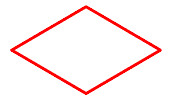Rhombuses (rhombii) have 4 equal sides. Both pairs of opposite sides are parallel. They all have 2 lines of symmetry (4 lines if they are a square!) All rhombuses belong to the parallelogram family. Parallelogram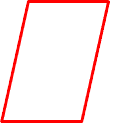Parallelograms have 2 pairs of parallel sides. Some parallelograms have lines of symmetry (depending on whether they are also squares, rectangles or rhombuses), but most do not. Trapezoid US (Trapezium UK)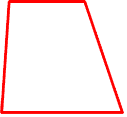Trapezoids US (Trapeziums UK) have one pair of parallel sides. Some trapezoids have a line of symmetry. Please note the differences between the definitions for US and UK. Kite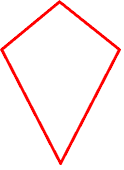Kites have 2 pairs of equal sides which are adjacent to each other. Trapezium US (Trapezoid UK)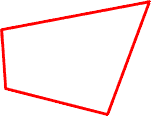Trapeziums US (Trapezoids UK) are quadrilaterals with no parallel sides. Please note the differences between the definitions for US and UK.

List of Geometric Shapes - Curved 2d Shapes

Here are some curved 2d shapes which have not yet been included.

 CircleCircles have a point in the centre from which each point on the diameter is equidistant. They have infinite lines of symmetry. How many sides does a circle have? This is an interesting question - the answer could be 0 (no straight sides), 1 curved side, or an infinite number of sides are all possible answers. EllipseEllipses are like circles which have been squashed or stretched. They have 2 lines of symmetry. They are also a special type of oval. The longest and shortest diameters of the ellipse are called the major and minor axes. These axes are also the lines of symmetry. CrescentCrescent shapes are made when two circles overlap, or when one circle is removed from another circle . The perimeter of crescents are made from two circular arcs. They have 1 line of symmetry. Our moon forms crescent shapes during its phases. Some countries such as Turkey or Algeria have crescent shapes on their flags.

List of Geometrics Shapes - 3D Shapes

 Cube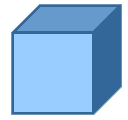Cubes have 6 faces, 12 edges and 8 vertices. All sides on a cube are equal length. All faces are square in shape. A cube is a type of cuboid. Cuboid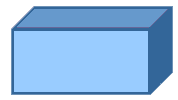Cuboids have 6 faces, 12 edges and 8 vertices. All the faces on a cuboid are rectangular. SphereSpheres have either 0 or 1 faces, 0 edges and 0 vertices. EllipsoidEllipsoids have either 0 or 1 faces, 0 edges and 0 vertices. Cylinder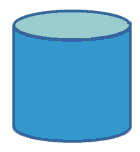Cylinders have either 2 or 3 faces, 0 or 2 edges, and 0 vertices. Cone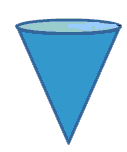Cones have either 1 or 2 faces, 0 or 1 edges, and 1 apex (which is described by some mathematicians as a vertex). Triangular Prism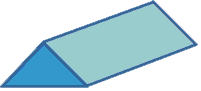Triangular Prisms have 5 faces, 9 edges, and 6 vertices. The two faces at either end are triangles, and the rest of the faces are rectangular. Hexagonal PrismHexagonal Prisms have 8 faces, 18 edges, and 12 vertices. The two faces at either end are hexagons, and the rest of the faces are rectangular. Triangular-based Pyramid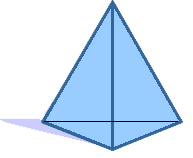Triangular-based pyramids have 4 faces, 6 edges and 4 vertices. The base is a triangle. All of the faces are triangular. If the triangular faces making up the prism are all equilateral, then the shape is also called a Tetrahedron. Square-based Pyramid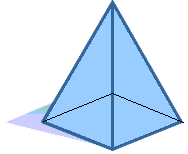Square based pyramids have 5 faces, 8 edges and 5 vertices The base is a square. All the other faces are triangular. Hexagonal Pyramid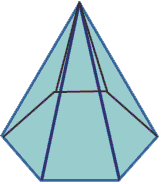Hexagonal pyramids have 7 faces, 12 edges, and 7 vertices. The base is a hexagon. All of the other faces are triangular.

The 5 Platonic Solids

The platonic solids form a set of 5 polyhedra with the following special properties:

• the faces of the platonic solids have to be regular and congruent.
• the same number of faces meet at each vertex.
 TetrahedronA Tetrahedrons is the same as a triangular pyramid. They have 4 triangular faces, 6 edges and 4 vertices. A regular tetrahedron has equilateral triangles for its faces, and is one of the 5 platonic solids. Cube (regular hexahedron)Cubes have 6 faces, 12 edges and 8 vertices. All sides on a cube are equal length. All faces are square in shape. A cube is a type of cuboid and is one of the 5 platonic solids. OctahedronOctahedrons are a shape with 8 faces, 12 edges and 6 vertices. A regular octahedron has equilateral triangles for its faces, and is one of the 5 platonic solids. DodecahedronDodecahedrons are a shape with 12 faces, 30 edges and 20 vertices. A regular dodecahedron has regular pentagons for its faces, and is one of the 5 platonic solids. IcosahedronIcosahedron are a shape with 20 faces, 30 edges and 12 vertices. All the faces are triangles. A regular icosahedron is one of the 5 platonic solids with all faces being equilateral triangles.

Short url:   http://multidict.net/cs/5437